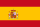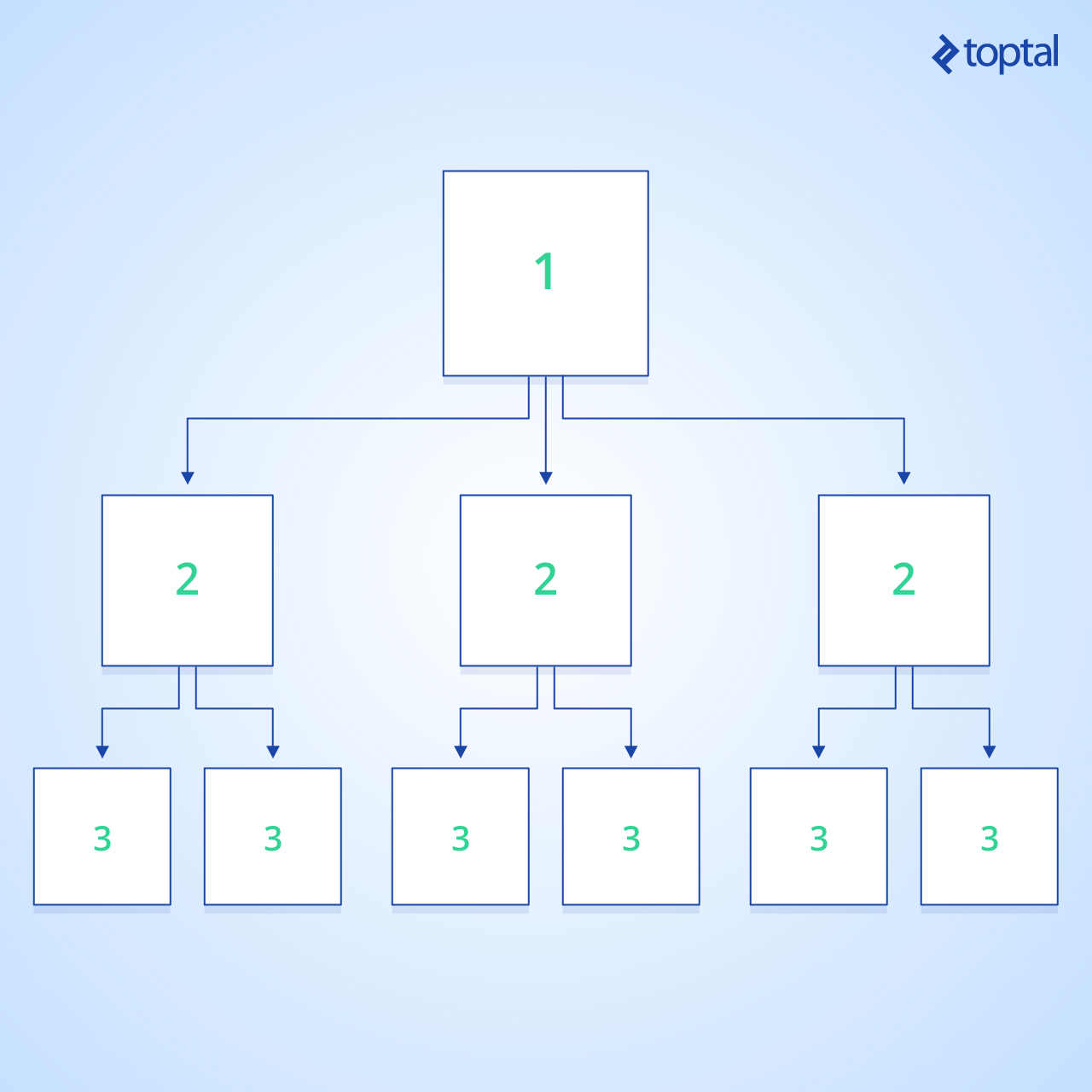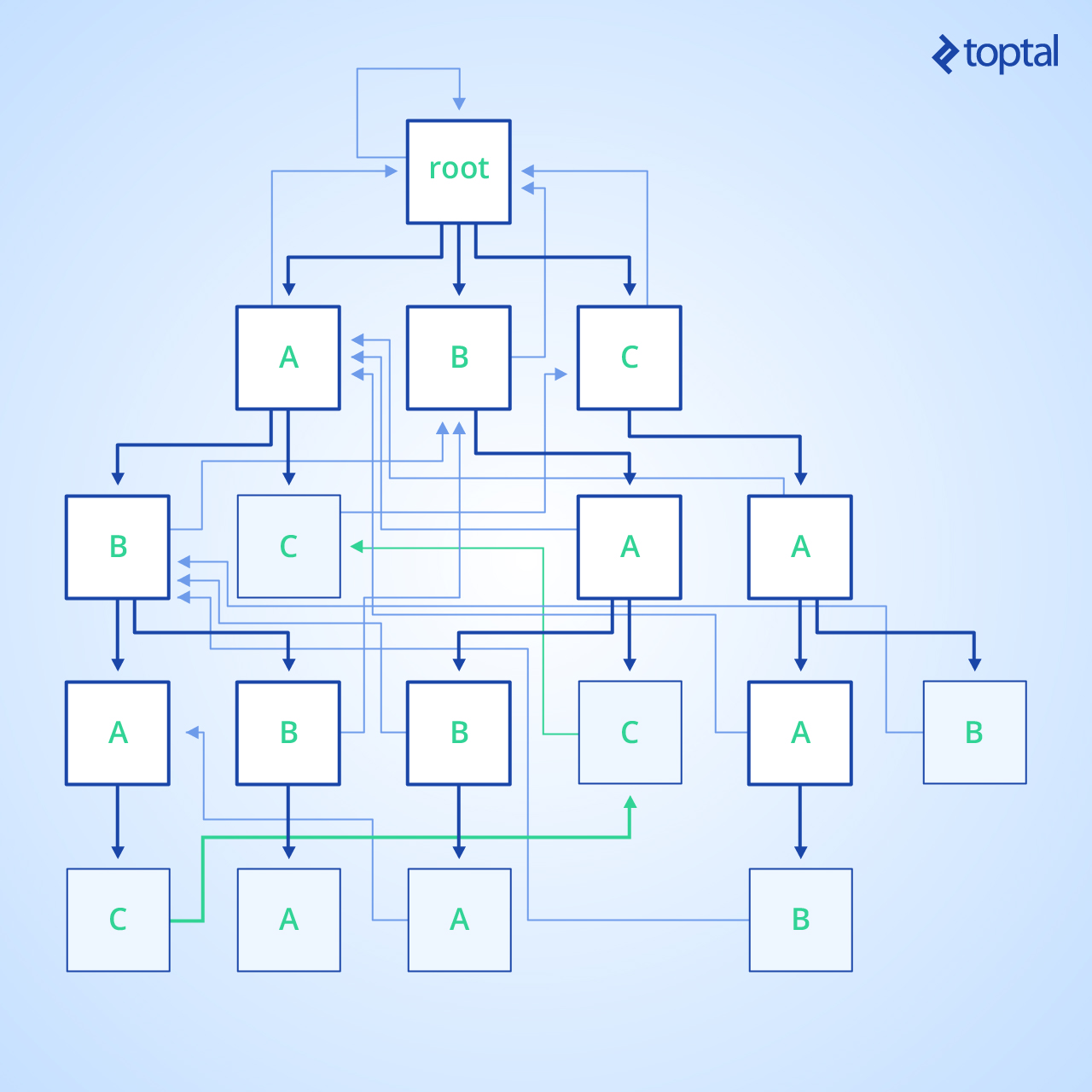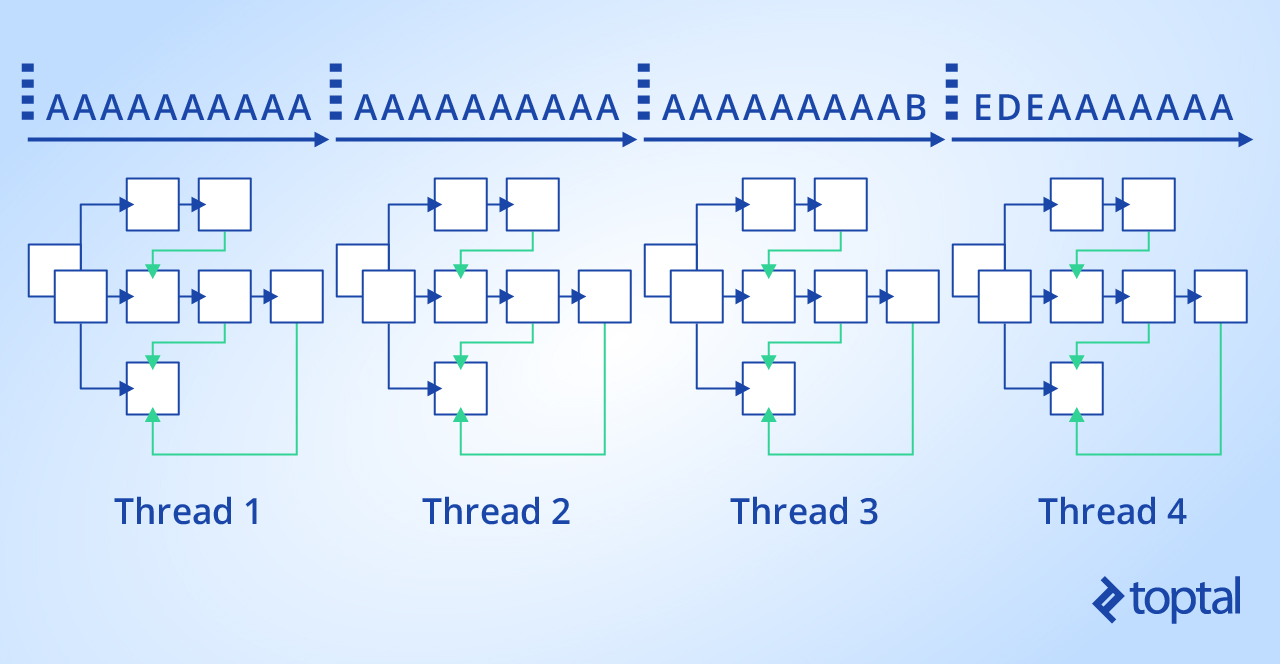Data Science and Databases

# Conquer String Search with the Aho-Corasick Algorithm

Roman is a SharePoint and .NET developer with a proven ability to develop efficient, scalable, and stable solutions for complex problems.
Read the Spanishversion of this article translated by Yesica Danderfer

Manipulating strings and searching for patterns in them are fundamental tasks in data science, and a typical task for any programmer.

Efficient string algorithms play an important role in many data science processes. Often they are what make such processes feasible enough for practical use.In this article, you will learn about one of the most powerful algorithms for searching for patterns in a large amount of text: The Aho-Corasick algorithm. This algorithm uses a trie data structure (pronounced “try”) to keep track of search patterns and uses a simple method to efficiently find all occurrences of a given set of patterns in any blob of text.

A previous article on the Toptal Engineering Blog demonstrated a string search algorithm for the same problem. The approach taken in this article offers improved computational complexity.

## The Knuth-Morris-Pratt (KMP) Algorithm

To understand how we can look for multiple patterns in a text efficiently, we need to first tackle an easier problem: Looking for a single pattern in a given text.

Suppose we have a large blob of text of length N and a pattern (that we want to look for in the text) of length M. Whether we want to look for a single occurrence of this pattern, or all of the occurrences, we can achieve a computational complexity of O(N + M) using the KMP algorithm.

### Prefix Function

The KMP algorithm works by calculating a prefix function of the pattern we are searching for. The prefix function pre-calculates a fallback position for every prefix of the pattern.

Let’s define our search pattern as a string, labeled `S`. For each substring `S[0..i]`, where `i >= 1`, we will find the maximum prefix of this string that also happens to be the suffix of this string. We’ll label the length of this prefix `P[i]`.

For the pattern “abracadabra,” the prefix function would produce the following fallback positions:

Index (`i`) 0 1 2 3 4 5 6 7 8 9 10
Character a b r a c a d a b r a
Prefix Length (`P[i]`) 0 0 0 1 0 1 0 1 2 3 4

The prefix function identifies an interesting characteristic of the pattern.

Let’s take a particular prefix of the pattern as an example: “abracadab.” The prefix function value for this prefix is two. This indicates that for this prefix “abracadab,” there exists a suffix of length two that matches exactly with the prefix of length two (i.e., the pattern starts with “ab” and the prefix ends with “ab.”) Furthermore, this is the longest such match for this prefix.

#### Implementation

Here is a C# function that can be used to calculate the prefix function for any string:

``````public int[] CalcPrefixFunction(String s)
{
int[] result = new int[s.Length]; // array with prefix function values

result = 0; // the prefix function is always zero for the first symbol (its degenerate case)
int k = 0; // current prefix function value
for (int i = 1; i < s.Length; i++)
{
// We're jumping by prefix function values to try smaller prefixes
// Jumping stops when we find a match, or reach zero
// Case 2 if we stop at a zero-length prefix
// Case 3 if we stop at a match
while (k > 0 && s[i] != s[k]) k = result[k - 1];
if (s[k] == s[i]) k++; // we've found the longest prefix - case 1
result[i] = k; // store this result in the array
}

return result;
}
``````

Running this function on a slightly longer pattern “abcdabcabcdabcdab” produces this:

Index (`i`) 0 1 2 3 4 5 6 7 8 9 10 11 12 13 14 15 16
Character a b c d a b c a b c d a b c d a b
Prefix Function (`P[i]`) 0 0 0 0 1 2 3 1 2 3 4 5 6 7 4 5 6

#### Computational Complexity

Despite the fact that there are two nested loops, the complexity of the prefix function is just O(M), where M is the length of pattern S.

This can be explained easily by observing how the loops work.

All outer loop iterations through `i` can be divided into three cases:

1. Increases `k` by one. The loop completes one iteration.

2. Doesn’t change the zero value of `k`. The loop completes one iteration.

3. Doesn’t change or decreases a positive value of `k`.

The first two cases can run at most M times.

For the third case, let’s define `P(s, i) = k1` and `P(s, i + 1) = k2, k2 <= k1`. Each of these cases should be preceded by `k1 - k2` occurences of the first case. The count of decreases does not exceed `k1 - k2 + 1`. And in total we have no more than 2 * M iterations.

#### Explanation of the Second Example

Let’s look at the second example pattern “abcdabcabcdabcdab”. Here is how the prefix function processes it, step by step:

1. For an empty substring and the substring “a” of length one, the value of the prefix function is set to zero. (`k = 0`)

2. Look at the substring “ab.” The current value of `k` is zero and the character “b” does not equal the character “a.” Here, we have the second case from the previous section. The value of `k` stays at zero and the value of the prefix function for substring “ab” is also zero.

3. It’s the same case for the substrings “abc” and “abcd.” There are no prefixes that are also the suffixes of these substrings. The value for them stays at zero.

4. Now let’s look at an interesting case, the substring “abcda.” The current value of `k` is still zero, but the last character of our substring matches with its first character. This triggers the condition of `s[k] == s[i]`, where `k == 0` and `i == 4`. The array is zero-indexed, and `k` is the index of the next character for the maximum-length prefix. This means that we have found the maximum-length prefix for our substring that is also a suffix. We have the first case, where the new value of `k` is one, and thus the value of the prefix function P(“abcda”) is one.

5. The same case also happens for the next two substrings, P(“abcdab”) = 2 and P(“abcdabc”) = 3. Here, we are searching for our pattern in the text, comparing strings character by character. Let’s say the first seven characters of the pattern matched with some seven consecutive characters of processed text, but on the eighth character it doesn’t match. What should happen next? In the case of naïve string matching, we should return seven characters back and start the comparison process again from the first character of our pattern. With the prefix function value (here P(“abcdabc”) = 3) we know that our three-character suffix already matches three characters of text. And if the next character in the text is “d,” the length of the matched substring of our pattern and substring in the text is increased to four (“abcd”). Otherwise, P(“abc”) = 0 and we will start the comparison process from the first character of pattern. But what is important is that we don’t return during the processing of the text.

6. The next substring is “abcdabca.” In the previous substring, the prefix function was equal to three. This means that `k = 3` is greater than zero, and at the same time we have a mismatch between the next character in the prefix (`s[k] = s = "d"`) and the next character in the suffix (`s[i] = s = "a"`). This means that we triggered the condition of `s[k] != s[i]`, and that the prefix “abcd” can’t be the suffix of our string. We should decrease the value of `k` and take the previous prefix for comparison, where possible. As we described above, the array is zero-indexed, and `k` is the index of the next character we check from the prefix. The last index of the currently matched prefix is `k - 1`. We take the value of the prefix function for the currently matched prefix `k = result[k - 1]`. In our case (the third case) the length of the maximum prefix will be decreased to zero and then in the next line will be increased up to one, because “a” is the maximum prefix that is also the suffix of our substring.

7. (Here we continue our calculation process until we reach a more interesting case.)

8. We start processing the following substring: “abcdabcabcdabcd.” The current value of `k` is seven. As with “abcdabca” above, we have hit a non-match: Because character “a” (the seventh character) doesn’t equal character “d,” substring “abcdabca” can’t be the suffix of our string. Now we get the already calculated value of the prefix function for “abcdabc” (three) and now we have a match: Prefix “abcd” is also the suffix of our string. Its maximum prefix and the value of the prefix function for our substring is four, because that’s what the current value of `k` became.

9. We continue this process until the end of the pattern.

In short: Both cycles take no more than 3 M iterations, which proves the complexity to be O(M). Memory use is also O(M).

### KMP Algorithm Implementation

``````public int KMP(String text, String s)
{
int[] p = CalcPrefixFunction(s); // Calculate prefix function for a pattern string

// The idea is the same as in the prefix function described above, but now
// we're comparing prefixes of text and pattern.

// The value of maximum-length prefix of the pattern string that was found
// in the text:
int maxPrefixLength = 0;
for (int i = 0; i < text.Length; i++)
{
// As in the prefix function, we're jumping by prefixes until k is
// greater than 0 or we find a prefix that has matches in the text.
while (maxPrefixLength > 0 && text[i] != s[maxPrefixLength])
maxPrefixLength = p[maxPrefixLength - 1];

// If a match happened, increase the length of the maximum-length
// prefix.
if (s[maxPrefixLength] == text[i]) maxPrefixLength++;

// If the prefix length is the same length as the pattern string, it
// means that we have found a matching substring in the text.
if (maxPrefixLength == s.Length)
{
// We can return this value or perform this operation.
int idx = i - s.Length + 1;

// Get the previous maximum-length prefix and continue search.
maxPrefixLength = p[maxPrefixLength - 1];
}
}

return -1;
}
``````

The above algorithm iterates through the text, character by character, and tries to increase the maximum prefix for both our pattern and some sequence of consecuitve characters in the text. In case of failure we will not return our position to earlier in the text. We know the maximum prefix of the found substring of the pattern; this prefix is also the suffix of this found substring and we can simply continue the search.

The complexity of this function is the same as that for the prefix function, making the overall computational complexity O(N + M) with O(M) memory.

Trivia: The methods `String.IndexOf()` and `String.Contains()` in the .NET framework have an algorithm with the same complexity under the hood.

## The Aho-Corasick Algorithm

Now we want to do the same for multiple patterns.

Suppose there are M patterns of lengths L1, L2, …, Lm. We need to find all matches of patterns from a dictionary in a text of length N.

A trivial solution would be taking any algorithm from the first part and running it M times. We have complexity of O(N + L1 + N + L2 + … + N + Lm), i.e. O(M * N + L).

Any serious enough test kills this algorithm.

Taking a dictionary with the 1,000 most common English words as patterns and using it to search the English version of Tolstoy’s “War and Peace” would take quite a while. The book is over three million characters long.

If we take the 10,000 most common English words, the algorithm will work around 10 times slower. Obviously on inputs greater than this one, execution time will also grow.

This is where the Aho-Corasick algorithm does its magic.

The complexity of the Aho-Corasick algorithm is O(N + L + Z), where Z is the count of matches. This algorithm was invented by Alfred V. Aho and Margaret J. Corasick in 1975.

### Implementation

Here, we need a trie, and we add to our algorithm a similar idea to the prefix functions described above. We will calculate prefix function values for the whole dictionary.

Each vertex in the trie will store the following information:

``````public class Vertex
{
public Vertex()
{
Children = new Hashtable();
Leaf = false;
Parent = -1;
WordID = -1;
}

// Links to the child vertexes in the trie:
// Key: A single character
// Value: The ID of vertex
public Hashtable Children;

// Flag that some word from the dictionary ends in this vertex
public bool Leaf;

// Link to the parent vertex
public int Parent;

// Char which moves us from the parent vertex to the current vertex
public char ParentChar;

// Suffix link from current vertex (the equivalent of P[i] from the KMP algorithm)

// Link to the leaf vertex of the maximum-length word we can make from the current prefix

// If the vertex is the leaf, we store the ID of the word
public int WordID;
}
``````

There are multiple ways of implementing child links. The algorithm will have a complexity of O(N + L + Z) in the case of an array, but this will have an additional memory requirement of O(L * q), where `q` is the length of the alphabet, since that is the maximum number of children a node can have.

If we use some structure with O(log(q)) access to its elements, we have an additional memory requirement of O(L), but complexity of the whole algorithm will be O((N + L) * log(q) + Z).

In the case of a hash table, we have O(L) additional memory, and the complexity of the whole algorithm will be O(N + L + Z).

This tutorial uses a hash table because it will also work with different character sets, e.g., Chinese characters.

We already have a structure for a vertex. Next, we will define a list of vertices and initialize the root node of the trie.

``````public class Aho
{
List<Vertex> Trie;
List<int> WordsLength;
int size = 0;
int root = 0;

public Aho()
{
Trie = new List<Vertex>();
WordsLength = new List<int>();
Init();
}

private void Init()
{
size++;
}
}
``````

Then we add all patterns to the trie. For this, we need a method to add a words to the trie:

``````public void AddString(String s, int wordID)
{
int curVertex = root;
for (int i = 0; i < s.Length; ++i) // Iterating over the string's characters
{
char c = s[i];

// Checking if a vertex with this edge exists in the trie:
if (!Trie[curVertex].Children.ContainsKey(c))
{

Trie[size].Parent = curVertex;
Trie[size].ParentChar = c;
Trie[curVertex].Children[c] = size;
size++;
}
curVertex = (int)Trie[curVertex].Children[c]; // Move to the new vertex in the trie
}
// Mark the end of the word and store its ID
Trie[curVertex].Leaf = true;
Trie[curVertex].WordID = wordID;
}
``````

At this point, all of the pattern words are in the data structure. This requires an additional memory of O(L).

Next we need to calculate all suffix links and dictionary entry links.

To make it clear and simple to understand, I’m going to traverse our trie from the root to the leaves and make similar calculations like we made for the KMP algorithm, but in contrast to the KMP algorithm, where we find the maximum-length prefix that also was the suffix of the same substring, now we will find the maximum-length suffix of the current substring that also is the prefix of some pattern in the trie. The value of this function will be not the length of the found suffix; it will be the link to the last character of the maximum suffix of the current substring. This is what I mean by the suffix link of a vertex.

I will process vertices by levels. For that, I will use a breadth-first search (BFS) algorithm:And below is the implementation of this traversal:

``````public void PrepareAho()
{
Queue<int> vertexQueue = new Queue<int>();
vertexQueue.Enqueue(root);
while (vertexQueue.Count > 0)
{
int curVertex = vertexQueue.Dequeue();

foreach (char key in Trie[curVertex].Children.Keys)
{
vertexQueue.Enqueue((int)Trie[curVertex].Children[key]);
}
}
}
``````

And below is the `CalcSuffLink` method for calculating the suffix link for each vertex (i.e. the prefix function value for each substring in the trie):

``````public void CalcSuffLink(int vertex)
{
// Processing root (empty string)
if (vertex == root)
{
return;
}
// Processing children of the root (one character substrings)
if (Trie[vertex].Parent == root)
{
return;
}
// Cases above are degenerate cases as for prefix function calculation; the
// value is always 0 and links to the root vertex.

// To calculate the suffix link for the current vertex, we need the suffix
// link for the parent of the vertex and the character that moved us to the
// current vertex.
char chVertex = Trie[vertex].ParentChar;
// From this vertex and its substring we will start to look for the maximum
// prefix for the current vertex and its substring.

while (true)
{
// If there is an edge with the needed char, we update our suffix link
// and leave the cycle
if (Trie[curBetterVertex].Children.ContainsKey(chVertex))
{
break;
}
// Otherwise, we are jumping by suffix links until we reach the root
// (equivalent of k == 0 in prefix function calculation) or we find a
// better prefix for the current substring.
if (curBetterVertex == root)
{
break;
}
}
// When we complete the calculation of the suffix link for the current
// vertex, we should update the link to the end of the maximum length word
// that can be produced from the current substring.
}
``````

The complexity of this method is O(L); depending on the implementation of the child collection, the complexity might be O(L * log(q)).

Proof of complexity is similar to the proof of complexity prefix function in the KMP algorithm.

Let’s look at the following image. This is a visualization of the trie for the dictionary `{ abba, cab, baba, caab, ac, abac, bac }` with all its calculated info:Trie edges are deep blue, suffix links are light blue, and dictionary suffix links in green. Nodes corresponding to dictionary entries are highlighted in blue.

And now we need only one more method—processing a block of text we intend to search through:

``````public int ProcessString(String text)
{
// Current state value
int currentState = root;

// Targeted result value
int result = 0;

for (int j = 0; j < text.Length; j++)
{
// Calculating new state in the trie
while (true)
{
// If we have the edge, then use it
if (Trie[currentState].Children.ContainsKey(text[j]))
{
currentState = (int)Trie[currentState].Children[text[j]];
break;
}
// Otherwise, jump by suffix links and try to find the edge with
// this char

// If there aren't any possible edges we will eventually ascend to
// the root, and at this point we stop checking.
if (currentState == root) break;
}
int checkState = currentState;

// Trying to find all possible words from this prefix
while (true)
{
// Checking all words that we can get from the current prefix

// If we are in the root vertex, there are no more matches
if (checkState == root) break;

// If the algorithm arrived at this row, it means we have found a
// pattern match. And we can make additional calculations like find
// the index of the found match in the text. Or check that the found
// pattern is a separate word and not just, e.g., a substring.
result++;
int indexOfMatch = j + 1 - WordsLength[Trie[checkState].WordID];

// Trying to find all matched patterns of smaller length
}
}

return result;
}
``````

And, now this is ready for use:

On input, we have a list of patterns:

``````List<string> patterns;
``````

And search text:

``````string text;
``````

And here’s how to glue it together:

``````// Init the trie structure. As an optional parameter we can put the approximate
// size of the trie to allocate memory just once for all nodes.
Aho ahoAlg = new Aho();

for (int i = 0; i < patterns.Count; i++)
{
}
// Prepare algorithm for work (calculates all links in the structure):
ahoAlg.PrepareAho();

// Process the text. Output might be different; in my case, it's a count of
// matches. We could instead return a structure with more detailed information.
int countOfMatches = ahoAlg.ProcessString(text);
``````

And that’s it! You now know how this simple-yet-powerful algorithm works!

Aho-Corasick is really flexible. Search patterns don’t have to be only words, but we can use whole sentences or random chains of characters.

### Performance

The algorithm was tested on an Intel Core i7-4702MQ.

For tests, I took two dictionaries: The 1,000 most common English words, and the 10,000 most common English words.

To add all these words to the dictionary and prepare the data structure to work with each of the dictionaries, the algorithm required 55ms and 135ms respectively.

The algorithm processed real books of 3-4 million characters in length within 1.0-1.3 seconds, while it took 9.6 seconds for a book with around 30 million characters.

## Parallelizing the Aho-Corasick Algorithm

Going parallel with the Aho-Corasick algorithm is not a problem at all:A large text can be divided into multiple chunks and multiple threads can be used to process each chunk. Each thread has access to the generated trie based on the dictionary.

What about words being split up at the boundary between chunks? This problem can be solved easily.

Let N be the length of our large text, S be the size of a chunk, and L be the length of the largest pattern in the dictionary.

Now we can use a simple trick. We divide chunks with some overlap at the end, for example taking `[S * (i - 1), S * i + L - 1]`, where `i` is index of the chunk. When we get a pattern match, we can easily get the start index of the current match and just check that this index is within the chunk range without overlaps, `[S * (i - 1), S * i - 1]`.

## A Versatile String Search Algorithm

The Aho-Corasick algorithm is a powerful string matching algorithm that offers the best complexity for any input and doesn’t require much additional memory.

The algorithm is often used in a various systems, such as spell checkers, spam filters, search engines, bioinformatics/DNA sequence searching, etc. In fact, some popular tools that you may be using every day are using this algorithm behind the scenes.

The prefix function from the KMP algorithm in itself is an interesting tool that brings the complexity of single-pattern matching down to linear time. The Aho-Corasick algorithm follows a similar approach and uses a trie data structure to do the same for multiple patterns.

I hope you have found this tutorial on the Aho-Corasick algorithm useful.